Related Book
Introduction to Stochastic Search and Optimization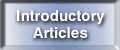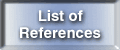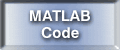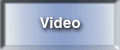Further information: James C. Spall Johns Hopkins University Applied Physics Laboratory 11100 Johns Hopkins Rd. Laurel, MD 20723-6099 USA(unique visitors)
Initialized on 29 March 2001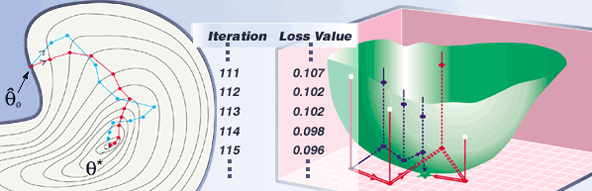A Method for System Optimization
 The Setting Selected Applications SPSA Overview Features of SPSA Relationship to Other Search and Optimization MethodsThe Setting

Individuals and organizations are often faced with making tradeoffs between different factors in order to achieve desirable outcomes. Choosing these tradeoffs in the "best" way is the essence of the optimization problem. Mathematical algorithms for search and optimization play a large role in finding the best options in many problems in engineering, business, medicine, and the natural and social sciences. Such algorithms start with an initial "guess" at a solution, and this estimated solution is updated on an iteration-by-iteration basis with the aim of improving the performance measure (objective function). In most practical problems, the solution depends on more than one quantity, leading to the multivariate optimization problem of minimizing or maximizing an objective function dependent on multiple variables. See the introductory article on stochastic optimization for a general introduction to issues in optimization in a stochastic setting.

There has recently been much interest in recursive optimization algorithms that rely on measurements of only the objective function to be optimized, not on direct measurements of the gradient (derivative) of the objective function. Such algorithms have the advantage of not requiring detailed modeling information describing the relationship between the parameters to be optimized and the objective function. For example, many systems involving human beings or computer simulations are difficult to treat analytically, and could potentially benefit from such an optimization approach. We now summarize an algorithm that is especially suited to such problems.

SPSA Overview                                    Top

One optimization method that has attracted considerable international attention is the simultaneous perturbation stochastic approximation (SPSA) method. As motivated above—and like methods such as simulated annealing or genetic algorithms—SPSA uses only objective function measurements. This contrasts with algorithms requiring direct measurements of the gradient of the objective function (which are often difficult or impossible to obtain). Further, SPSA is especially efficient in high-dimensional problems in terms of providing a good solution for a relatively small number of measurements of the objective function.

The essential feature of SPSA, which provides its power and relative ease of use in difficult multivariate optimization problems, is the underlying gradient approximation that requires only two objective function measurements per iteration regardless of the dimension of the optimization problem. These two measurements are made by simultaneously varying in a "proper" random fashion all of the variables in the problem (the "simultaneous perturbation"). This contrasts with the classical ("finite-difference") method where the variables are varied one at a time. If the number of terms being optimized is p, then the finite-difference method takes 2p measurements of the objective function at each iteration (to form one gradient approximation) while SPSA takes only two measurements. A fundamental result on relative efficiency then follows:

 Under reasonably general conditions, SPSA and the standard finite-difference SA method achieve the same level of statistical accuracy for a given number of iterations even though SPSA uses p times fewer measurements of the objective function at each iteration (since each gradient approximation uses only 1/p the number of function measurements). This indicates that SPSA will converge to the optimal solution within a given level of accuracy with p times fewer measurements of the objective function than the standard method.

An equivalent way of interpreting the statement above is the following:

 One properly generated simultaneous random change of all p variables in the problem contains as much information for optimization as a full set of p one-at-a-time changes of each variable.

Further, SPSA—like other stochastic approximation methods—formally accommodates noisy measurements of the objective function. This is an important practical concern in a wide variety of problems involving Monte Carlo simulations, physical experiments, feedback systems, or incomplete knowledge.

Selected Applications
Top

Some of the general areas for application of SPSA include statistical parameter estimation, simulation-based optimization, pattern recognition, nonlinear regression, signal processing, neural network training, adaptive feedback control, and experimental design. Specific system applications represented in the list of references include

 Adaptive optics Aircraft modeling and control Atmospheric and planetary modeling Cardiological data analysis Circuit design Economic or defense policymaking Fault detection in plant operations Human-machine interface control Industrial quality improvement Medical imaging Noise cancellation Process control Queuing network design Robot control Sensor placement Traffic signal timing or other transportation problems Underground mine detection Wastewater treatment

Features of SPSA
Top

SPSA has several features that make it attractive for many practical applications, such as the ones mentioned above.

1. Because of the efficient gradient approximation, the algorithm is appropriate for high-dimensional problems where many terms are being determined in the optimization process. Many practical applications have a significant number of terms to be optimized.
2. SPSA allows for the input to the algorithm to be measurements of the objective function corrupted by noise. For example, this is ideal for the case where Monte Carlo simulations are being used because each simulation run provides one noisy estimate of the performance measure. This is especially relevant in practice as a very large number of scenarios often need to be evaluated, and it will not be possible to run a large number of simulations at each scenario (to average out the noise). So, an algorithm explicitly designed to handle noise is needed.
3. Performance guarantees for SPSA exist in the form of an extensive convergence theory. The theory pertains to both local optimization (Spall, 1992) and global optimization in the face of multiple local optima (Maryak and Chin, 2008) and fully allows for noisy values of the objective function. The algorithm has desirable properties for both global and local optimization in the sense that the gradient approximation is sufficiently noisy to allow for escape from local minima while being sufficiently informative about the slope of the function to facilitate local convergence. This may avoid the cumbersome need in many global optimization problems to manually switch from a global to a local algorithm.
4. Implementation of SPSA may be easier than other stochastic optimization methods (such as forms of the genetic algorithm) since there are fewer algorithm coefficients that need to be specified, and there are some published guidelines providing insight into how to pick the coefficients in practical applications (Spall, 1998). (This is not to imply that a serious implementation of SPSA to a difficult problem will be easy. Certainly, some "trial and error" experimentation will be required for an effective implementation. No general optimization method can avoid this.)
5. While the original SPSA method is designed for continuous optimization problems, there have been recent extensions to discrete optimization problems (Gerencsér, et al., 2004, and Hill, 2005). This may be relevant to certain design problems, for example, where one wants to find the best number of items to use in a particular application.
6. While "basic" SPSA uses only objective function measurements to carry out the iteration process in a stochastic analogue of the steepest descent method of deterministic optimization, it is also possible to have efficient stochastic analogues of the famous Newton-Raphson algorithm from deterministic optimization (which uses gradients and Hessian [second derivative] matrices of the objective function). This extension is the adaptive SPSA algorithm in Spall (2000) and Spall (2009), which builds an estimate of the Hessian matrix from either (noisy) measurements of the loss function or, if available, from direct (noisy) measurements of the gradient of the loss function.
7. Formal theoretical and numerical algorithmic comparisons of SPSA with other state-of-the-art optimization methods (simulated annealing, evolutionary computation, etc.) have generally shown SPSA to be competitive (and possibly more efficient) in terms of the overall cost of the optimization process (Spall, et al., 2006, and the list of references ). This is especially the case when only noisy values of the objective function are available.

The introductory article on SPSA and the list of references provide a more-detailed sense of the performance of SPSA and the type of problems for which SPSA is appropriate.                                   Top

Relationship to Other Search and Optimization Methods

There are a large number of methods for numerical optimization in multivariate problems. Hence, a user with a challenging optimization problem faces the daunting task of determining which algorithm is appropriate for a given problem. This choice is made more difficult by the large amount of "hype" and dubious claims that are associated with some popular algorithms. An inappropriate approach may lead to a large waste of resources, both from the view of wasted efforts in implementation and from the view of the resulting suboptimal solution to the optimization problem of interest.

No search algorithm is uniformly better than all other algorithms across all possible problems. It is clear, however, that some algorithms may work better than others on certain classes of problems as a consequence of being able to exploit the problem structure. For example, traditional nonlinear programming methods (e.g., constrained conjugate gradient) are well suited to deterministic optimization problems with exact knowledge of the gradient of the objective function. Methods involving a population of candidate solutions, such as genetic algorithms, may be useful for a broad search over the domain of the parameters being optimized and subsequent initialization of more powerful local search algorithms. (Simple random search may also be useful for such a "crude" search if it is not desirable or feasible to work with a population of solutions.) Standard backpropagation may be effective in certain neural network applications when it is possible to calculate the required gradient of the objective function. More generally, stochastic gradient methods (i.e., Robbins-Monro stochastic approximation) are effective if one has direct (unbiased) measurements of the gradient of the objective function.

SPSA is generally used in nonlinear problems having many variables where the objective function gradient is difficult or impossible to obtain. ("Many" here is a relative concept. In some problems, this may represent five or ten variables; in other problems, this may represent thousands of variables or more.) As a stochastic approximation algorithm, SPSA may be rigorously applied when noisy measurements of the objective function are all that are available. (Noisy measurements can have a profound degrading effect on algorithms that are not designed to cope with noise. Noise may prevent convergence or may dramatically decrease efficiency in such algorithms.) There have also been many successful applications of SPSA in settings where perfect (noise-free) measurements of the loss function are available.

In summary, SPSA is a powerful method for optimization in challenging nonlinear problems. It has a strong theoretical foundation and is often more efficient and easier to implement than well-known methods such as simulated annealing or genetic algorithms. Many examples of the use of SPSA are given in the list of references.

Key words related to SPSA: Optimization; parameter estimation; stochastic approximation; root-finding; random directions; Kiefer-Wolfowitz stochastic approximation; Robbins-Monro stochastic approximation; simultaneous perturbation; adaptive estimation; stochastic gradient; stochastic programming; stochastic optimization.
Top